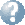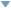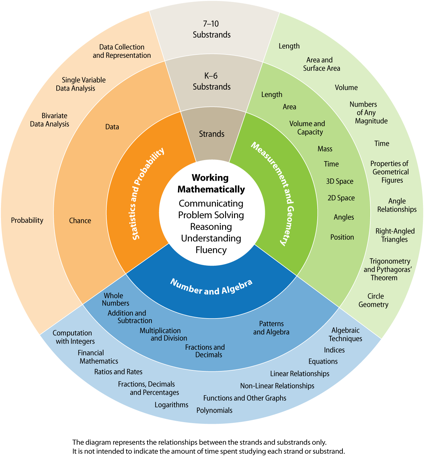Online Reference Dictionary, Encyclopedia & moreby: WordStarts with Ends withMentionsteaching:topics:thinking_mathematically:problemsolving:summary

## being systematic about solving problems

This is relevant from primary school all the way through high school and university and beyond, finding solutions in all kinds of professional tasks — science, business, design, engineering, computers, policy making and management — pretty much any field which requires thought and initiative rather than simply carrying out repetitive set actions. It is one of the ways we analyse and talk about the world.It is also at the centre of the maths syllabus, every year from infants to year 12.

If you are in primary school … some of the language I am using here is talking to older students, don’t worry about that — skip those parts — you have plenty of time to learn about that stuff later.

Just have a go, jump into one of the easier problems in this collection: problemsolvingwall200.pdf — they go from primary school to senior high school level — find one you think might be interesting.

If you are further along this journey dig a little deeper. Return to it later, much of the maths you are studying is related to these ideas. By the time you leave school I hope you have a strong sense of how to approach a problem more formally, how to use a mathematical model to understand the relationships and find a solution.

### a summary of the main ideas

#### What exactly is being asked?

• what is the problem?
• why are you being asked to solve it?
• in a school test: so that you can show that you understand the ideas that you have been taught.
• it is very easy to jump into answering something else entirely!
• you might have to dig a little to find the actual question that needs answering.

#### What form of answer is called for?

• in a test — make sure you get this from the question!
• in life — the context, and the audience, suggests the kind of response required.
• in each of these the argument backing your result is the main part of your answer.
• is a number (or numbers) required?
• for measurements — what are the units?
• an exact value? — hence a fraction or surd — this is the ‘default’
• do you also need an approximate value? — a decimal — how accurate is the data given?
• give it (them) a name — a pronumeral, it is the most important ‘unknown’!
• is a diagram, picture or some graphical representation required?
• away from the very contrived exam or test context your answer must show the relationships that you found in a form that your audience can read — maths is very much about communicating particular kinds of abstract ideas.
• in school, and in every other application of maths, it is the argument, the reasoning, that is important — the justification for your conclusions, the reason someone should believe what you are saying.
• we say “Show your working!!” — what this means is explain how you got your answer, make a clear argument that someone else could follow (someone who already understands the topic, and the notation you are using, and who is familiar with the rules you have covered in class)

#### What is required to find this value? (or values, or details in a diagram or graph)

• name any intermediate values required … draw pictures to help understand relationships!
• write down any equations connecting these with each other or the answer (or any step toward it!)
• finding the relationships between the facts given is the path to every answer.

#### What facts have you been given?

• write them down as equations, or draw them
• then recheck, does what you wrote fit the information given — is it sensible?
• there might be some extra, unimportant, facts given
• as you work on the problem, keep going back to the question
• some facts might be a bit hidden
• some later phrase might clarify what an earlier one said
• some algebra might reveal something is impossible, or fixed, and that can resolve issues about what the question really meant, perhaps clear up ambiguities
• consider each phrase in the question — break the question into the different ‘facts’ presented
• then write equations for relationships between the values given in these phrases
• find any facts given in pictures, graphs or diagrams
• lengths, distances, areas or other measurements labelled with values, units or names
• angles labelled with values or names … look for any right angles and parallel lines.
• note any shapes, angles or lengths that are equivalent or described
• fill in details …
• are any shapes shown also described in the question? what are you told about them!
• label points fully … egquad(2,5)quadorquad(a,0)
• label axes, directions and scales
• label lines on graphs with their equations
• thoughtfully and succinctly marking these names and labels will help understand the problem
• this step often shows a path to a solution
• draw diagrams, or label the ones given, with any facts you have been told
• look for geometrical facts connecting the values (known or named) in any diagram
• if you are looking for angles … think parallel lines and other angle rules
• if you are looking for distances … think pythagoras, similar triangles, sides of shapes and other rules about length
• if the problem mixes angles and lengths trigonometry ratios might be important
• write down any relationship between what you are given and the unknowns you have named (you have probably already done most of this, if you have been careful so far)
• perhaps some digging will be required, to find more ‘facts’ or discover other relationships
• perhaps some facts are hidden in the diagrams or the rules of geometry?
• perhaps you need to use some known laws or properties, especially in science questions or topics with specific methods you learnt
• be familiar with where to find formula you have been shown (see the notes about formula sheets below in a test, or know were reference material is — in your textbooks or online)

#### Combine these facts and relationships

• here is the main algebra part
• substitute known properties of variables into equations to find new facts, new equations
• combine two equations, two facts, to find a new one with fewer variables
• logically you are trying to eliminate some variables to find relationships between the others
• then step by step get a particular value for one of the ‘unknowns’
• then use that value to find the other values using earlier ‘facts’
• this process requires creative manipulation of the different equations
• this stage is the real ‘thinking’ part, using mathematics to solve problems.
• often there is a step that requires creative insight, that is not obvious at first
• if you are revising or studying a topic — put this step into your notes
• always keep the goal in mind
• often we are trying to find ways to get the equations into a particular ‘form’
• we want one that will show us the answer the question calls for.
• explore, try things out — play around with the equations
• you can’t break anything by filling up some blank paper!
• try to eliminate ‘unknowns’ from equations
• aim for an equation with just one ‘unknown’
• then that value can be found
• substitute it into previous equations
• then try again, with fewer unknowns
• the problem is now a bit smaller, you are getting closer to an answer

it is all about being systematic —

assembling what you know, expressing it clearly and succinctly, remembering some rules, doing a bit of manipulation

then giving an answer to the actual questionalways in an appropriate form!

• are the values found physically or logically plausible?
• do the values found actually fit all the given facts? check each phrase!

Remember: if the question has lots of parts some information will only be needed for one little part (or not at all).

### problem solving in tests

• problem questions are often worth more than one mark, and usually each step is a half-mark or so.
• make sure you show the person marking everything that you think may be important, and the reasons you made each step in your working … this is basic exam strategy, the marker cannot read your mind.
• if you do not get the full answer you will still get marks for getting half way …
• but only if you show clearly that you did at least understand the problem
• even better — show that you are part of the way to the solution!
• if you are running out of time … try outlining the steps you need to take, this can be much faster than working out the details, and it might get most of the marks, but only if very clearly described!
• if you are stuck and you have written down all facts you see that are relevant
• this will get you some marks if they are the right facts, if you are part way toward a solution
• it also allows you to move on and come back later, picking up where you left off
• maybe the next time you look at it you will see the way forward
• maybe some other question helps you understand or remember something
• maybe just thinking about something else lets your mind find the trick you need, the next step, in the background

#### formula sheets

• are in every exam — make sure, during revision, that you are familiar with the one you will be given
• where to find each formula
• the ‘standard’ uses of each one — the sheet gives you a big hint about which ‘standard’ methods might be asked!!
• make sure you know what variables and constants the symbols in these formula represent — they are given in the sheet with very minimal explanation (the title can help!)
• the idea is you are supposed to understand what they represent, what they show, how they are used — these sheets just help you remember the details
• there are some simple formula you are expected to know, that are not on the sheet.
• you can use rules, results established in earlier questions
• remember that you might use the answer to a previous part, even if you could not show it!
• this becomes more important as you move through the years … you will start to get multi-part questions that might be ‘show blah blah is true if’ and in the next part you need use that fact.